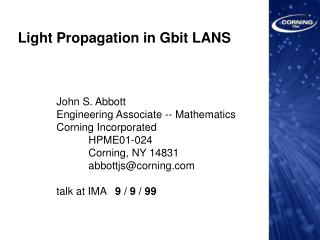# Light Propagation in Gbit LANS - PowerPoint PPT PresentationDownload PresentationLight Propagation in Gbit LANS

Light Propagation in Gbit LANS
Download Presentation## Light Propagation in Gbit LANS

- - - - - - - - - - - - - - - - - - - - - - - - - - - E N D - - - - - - - - - - - - - - - - - - - - - - - - - - -
##### Presentation Transcript

1. Light Propagation in Gbit LANS John S. Abbott Engineering Associate -- Mathematics Corning Incorporated HPME01-024 Corning, NY 14831 abbottjs@corning.com talk at IMA 9 / 9 / 99

2. Outline 1. Problem characterizing high speed lasers predicting performance of links 2. Background for multimode fibers 3. Measurement data 4. Modeling results 5. Review of Problem

3. Introduction To predict the performance of a link using multimode fiber, we need to know properties of both fiber and laser. The fiber’s properties (mode delays) derive from the measured index profile. The laser’s properties (modal power distribution) are equally important and need to be estimated.

4. The problems of interest 1. Predict the modal power distribution P_m in a 100-500m length fiber for a given laser -- for example, from the nearfield intensity I(r ) on a short length. 2. Show that this can be used to predict the bandwidth and performance of a multimode fiber given its measured index profile n(r ). 3. Determine limitations to this approach or better methods

5. Definition/Measurement of Bandwidth The bandwidth is the frequency where the FFT of the output pulse drops to 1/2 of its 0 frequency value. BW depends on mode delays & modal power

6. Laser BW = Source + FiberThe bandwidth depends on both laser and fiber. Different Lasers Different Fibers

7. Laser BW = Source + Fiber (TIA2.2) Different Lasers Different Fibers

8. Possible limitations 1. The intensity distribution I(r ) on a short length of fiber may not yet have settled into an excitation of individual propagating modes. Some think a continuum of modes is initially propagated and that these gradually evolve through destructive interference into a finite number of propagating modes. 2. Related modal noise/mode interaction effects -- current measurement technique is to ‘shake’ the fiber under test and average over time to reduce effects of modal interference.

9. One approach (JSA 1998 ) 1. Assume the intensity distribution I(r ) is given by 2. For a measured I(r ), determine the modal power distribution P_m which best matches I(r ) [in a least squares sense]. 3. Comparison with experiments on short fibers is somewhat encouraging but not satisfactory

10. Example Results -- least squares Intensity I(r ) (parabolic distribution) Calculated MPD (equal power)

11. Example Results -- least squares(using real data) Intensity data Calculated MPD

12. Example Results -- least squares(using real data -- example 2) Calculated MPD (negative value?) Intensity data

13. Second approach 1. Assume the intensity distribution I(r ) is due to a Gaussian beam with offset x and radius r, for which both I(r ) and P_m can be calculated explicitly. Again assume 2. For a measured I(r ), determine the best fit offset Gaussian beam(r,x) whose P_m best matches I(r ) [say in a least squares sense].

14. Calculation of Multimode Bandwidth The low frequency response of the system can be modeled as the sum of delta functions corresponding to the modes or mode groups: where (laser) (fiber) Thus the important parameters are

15. Solving for tau_m’s The light propagation can be approximated by the scalar wave equation, just as for single mode fiber: where is an eigenvalue and

16. Calculation of Multimode Bandwidth (2) The Fourier Transform of a sum of Delta functions is easy to do: So the amplitude is given by

17. Calculation of tau_m = mode delay by Perturbation Method(Olshansky/Nolan/Abbott/Button) Propagation Parameter Mode Delay (PMD)

18. Application of modeling mode delays -- DMD measurement

19. Conclusion To predict the performance of a link using multimode fiber, we need to know properties of both fiber and laser. The fiber’s properties (mode delays) derive from the measured index profile. The laser’s properties (modal power distribution) are equally important and need to be estimated.